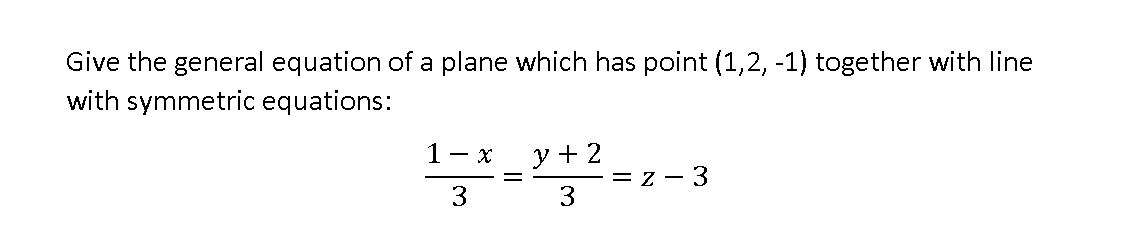# Give the general equation of a plane which has point (1,2, -1) together with line with symmetric equations: - x y + 2 3 = z - 3 3

Question

Giving the general equation of a line and plane.help_outlineImage TranscriptioncloseGive the general equation of a plane which has point (1,2, -1) together with line with symmetric equations: - x y + 2 3 = z - 3 3 fullscreen

### Want to see this answer and more?

Experts are waiting 24/7 to provide step-by-step solutions in as fast as 30 minutes!*

*Response times may vary by subject and question complexity. Median response time is 34 minutes for paid subscribers and may be longer for promotional offers.
Tagged in
Math
Calculus

### Other